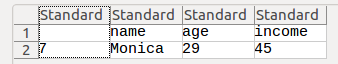R provides functions to read and write to various file formats. In this R Tutorial, we shall look specifically into CSV Files.

## R CSV Files

We shall learn R functions to :

Example of a CSV File, that we use in the examples going forward, is given below :

You may refer R Working Directory to modify the path of R Workspace to point to the directory containing your input files (CSV Files).

CSV Files are those files with values separated by commas in each row. Each row corresponds to a record or observation.

Syntax of function to read CSV File in R programming language :

Example to read CSV File in R programming language :

Please observe that the data of csv file is read to an R Data Frame.

## Process data read from CSV Files

R programming language reads the CSV File to an R Dataframe. So, you may use all the R Data Frame  functions to process the data.

Some of the examples are given below.

### Example R program to retrieve rows based on a condition applied to column

From the csv file, we shall extract rows, whose income is equal to the maximum of income.

## Write transformed data to CSV Files

Once we extract the required data or transform the data which is in data frame, we may write data frame to a CSV File.

### Example R program to write an R data frame to CSV File

We shall use the above example, where we extracted rows with maximum income, and write the resulting rows to a CSV File.### Conclusion :

In this R Tutorial – R CSV Files, we have learnt to read CSV File using R, process data read from CSV using R and write process data to CSV using R.